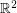# Inscribed Square Problem

 Importance: Medium ✭✭
 Author(s): Toeplitz
 Subject: Topology
 Keywords: simple closed curve square
 Posted by: dlh12 on: April 10th, 2008
Conjecture   Does every Jordan curve have 4 points on it which form the vertices of a square?

A Jordan curve is a continuous functionfrom the closed intervalto the planewith the properties thatis injective on the half-open interval(i.e.,is simple) and(i.e.,is closed).

## Bibliography

[M] Meyerson, M.D., Equilateral triangles and continuous curves, Fund. Math. 110, (1980), 1--9.

* indicates original appearance(s) of problem.

This was proven in https://arxiv.org/pdf/2005.09193.pdf

### inscription of squares in simple closed curves

There is a theorem that says:

in all simple closed curves there are 4n points that are vertex of n squares (inf = > n > =1)

Jorge Pasin.

### in all simple closed curves there are 4n points

Would you clarify? An obtuse triangle has only one inscribed square, so this theorem is not true for n>=2. Do you have a reference to this theorem? Strashimir Popvassilev

### Is the conjecture known to

Is the conjecture known to be true for C^1-smooth curves?

### Yes

Yes. Walter Stromquist, Inscribed squares and square-like quadrilaterals in closed curves, Mathematika 36: 187-197 (1989).

### no.

If it were true for C^1 curves, then since a Jordan curve is compact, it may be weierstrass approximated by a series of C^1 curves (indeed by curves whose component functions are polynomials) such that the series converges uniformly to the given jordan curve. Then by assumption, each curve in the sequence contains 4 points forming a square, and the sequence of squares can be regarded as (eventually) a sequence in the (sequentially) compact space of the 4-fold product of any closed epsilon enlargement of the area bounded by the original jordan curve. It follows that the sequence of squares contains a convergent subsequence, which can be shown to be a square lying on the original jordan curve.

Thus, proving the C^1 case proves the general case.

### This is flawed

The approximation argument is flawed: the squares on approximating curves may have sides decreasing to 0, in which case the limiting "square" degenerates to a point. In fact, Stromquist's theorem covers a much wider class of curves than C^1, but not all continuous curves.

### Quantifier

Phrasing should be changed from "Does any..." to "Does every..."